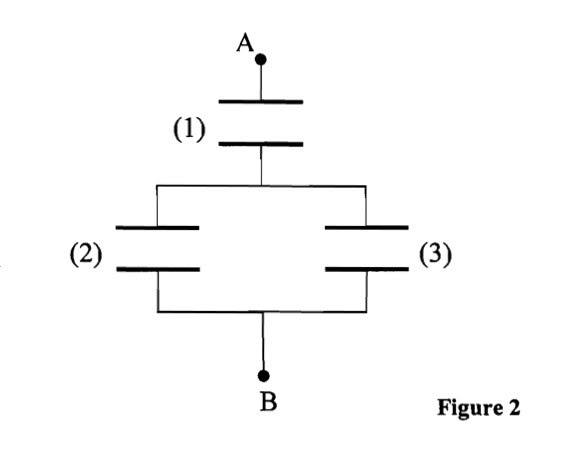# Energy of a Capacitor System with partially inserted Dielectric

## Homework StatementThe above is a capacitor system. A dielectric is inserted into capacitor C3 and I need to find the total energy of the system. The dielectric is not fully in, only partially (lets say a length x).

## The Attempt at a Solution

I first found the capacitance of C3 with the dielectric partially inserted. I know that dielectric makes a system of 2 parallel capacitors.

Hence

$$C3 = \frac{L\epsilon_{0}}{d} (x(\epsilon_{r}-1) + L)$$

where L is the length and width of the parallel capacitor.

Total capacitance of the system = $$\frac{(C3 + C2)C1}{C3 + C2 + C1}$$

To find the total energy, I use the formula $$1/2 \times \frac{Q^{2}}{C}$$

which becomes:

$$1/2 Q^{2} \times \frac{C3 + C2 + C1}{(C3 + C2)C1}$$

Does this seem correct? The answer give to me by the professor is:

$$U =1/2 \frac{Q^{2}}{C1} + \frac{Q^{2}}{C2 + C3}$$

I'm just kinda whether I'm wrong or the prof is...

Homework Helper
Hi saad87!$$1/2 Q^{2} \times \frac{C3 + C2 + C1}{(C3 + C2)C1}$$

Does this seem correct? The answer give to me by the professor is:

$$U =1/2 \frac{Q^{2}}{C1} + \frac{Q^{2}}{C2 + C3}$$

I'm just kinda whether I'm wrong or the prof is...

erm… they're the same! (assuming the professor's "1/2" is for both bits)1/(C2 + C3) = C1/(C2 + C3)C1